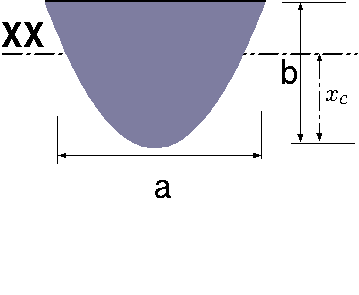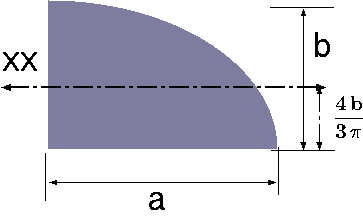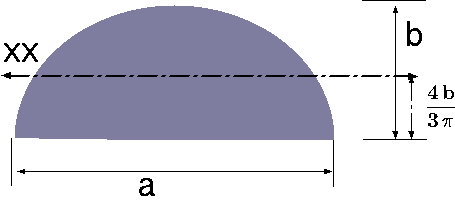Shape Name Picture Description $$\mathbf{x_c}$$, $$\mathbf{x_c}$$ $$\mathbf{A}$$ $$\mathbf{I_{xx}}$$ Rectangle$$\dfrac{a}{2}\,;\dfrac{b}{2}$$ $$a\,b$$ $$\dfrac{a\,b^{3}}{12}$$ Triangle$$\dfrac{a}{3}$$ $$\dfrac{a\,b}{3}$$ $$\dfrac{a\,b^{3}}{36}$$ Circle$$\dfrac{b}{2}$$ $$\dfrac{\pi\, b^2}{4}$$ $$\dfrac{\pi b^4}{64}$$ Ellipse$$\dfrac{a}{2}\; \dfrac{b}{2}$$ $$\dfrac{\pi\, ab}{4}$$ $$\dfrac{ab^2}{64}$$ $$y=\alpha\,x^2$$ Parabola$$\dfrac{3\,\alpha\,b}{15\,\alpha-5}$$ $$\dfrac{6\alpha -2}{3} \, \left( \dfrac{b}{\alpha}\right)^{\dfrac{3}{2}}$$ $$\dfrac{\sqrt{b}\,\left( 20\,{b}^{3}-14\,{b}^{2}\right) }{35\,\sqrt{\alpha}}$$ Quadrant of Circle$$\dfrac{4\,r}{3\,\pi}$$ $$\dfrac{\pi\,r^2}{4}$$ $$r^4\left(\dfrac{\pi}{16} - \dfrac{4}{9\pi}\right)$$ Ellipsoidal Quadrant$$\dfrac{4\,b}{3\,\pi}$$ $$\dfrac{\pi\,a\,b}{4}$$ $$a\,b^3\left(\dfrac{\pi}{16} - \dfrac{4}{9\pi}\right)$$ half of of Ellipse$$\dfrac{4\,b}{3\,\pi}$$ $$\dfrac{\pi\,a\,b}{4}$$ $$a\,b^3\left(\dfrac{\pi}{16} - \dfrac{4}{9\pi}\right)$$ Circular Sector$$0$$ $$2{\alpha}\,r^2$$ $$\dfrac{r^4}{4} \left(\alpha - \dfrac{1}{2}\sin2\alpha\right)$$ Circular Sector$$\dfrac{2}{3}\dfrac{r\,\sin\alpha}{\alpha}$$ $$2{\alpha}\,r^2$$ $$I_{x^{'}x^{'}} =$$$$\dfrac{r^4}{4} \left(\alpha + \dfrac{1}{2}\sin2\alpha\right)$$